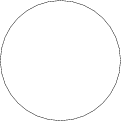Warning: unlink(/var/www/html/images/tmp/607fe2157901eb68e2d8c3d06c10d1cc.asy): No such file or directory in /var/www/html/extensions/Asymptote.php on line 109

Warning: unlink(/var/www/html/images/tmp/ltx-607fe2157901eb68e2d8c3d06c10d1cc.aux): No such file or directory in /var/www/html/extensions/Asymptote.php on line 113

Warning: unlink(/var/www/html/images/tmp/ltx-607fe2157901eb68e2d8c3d06c10d1cc.log): No such file or directory in /var/www/html/extensions/Asymptote.php on line 114

Warning: unlink(/var/www/html/images/tmp/607fe2157901eb68e2d8c3d06c10d1cc.eps): No such file or directory in /var/www/html/extensions/Asymptote.php on line 115

Warning: unlink(/var/www/html/images/tmp/607fe2157901eb68e2d8c3d06c10d1cc.log): No such file or directory in /var/www/html/extensions/Asymptote.php on line 116
User:Ulf Rehmann/Test2 - Encyclopedia of Mathematics

# User:Ulf Rehmann/Test2

Code (1)

\begin{equation}
\mu (B)= \sup \{\mu(K): K\subset B, \mbox{ $K$ compact} \}\,
\end{equation}


produces (but see below!):

\begin{equation} \mu (B)= \sup \{\mu(K): K\subset B, \mbox{ '"UNIQ-MathJax9-QINU"' compact} \}\, \end{equation}

as compared to the code (2):

\begin{equation}
\mu (B)= \sup \{\mu(K): K\subset B, K \mbox{ compact} \}\,
\end{equation}


which produces:

\begin{equation} \mu (B)= \sup \{\mu(K): K\subset B, K \mbox{ compact} \}\, \end{equation}

Interestingly, if code (1) is put inside $...$, it renders correctly, however it does not so, if it is put inside $...$.

$$e^{2\pi i} - 1 = 0$$

$$A,B,C, D, E, F, G$$

XXXYYY Additional text

Additional text $$(a+b)$$ $$(x-y)$$

no [[wiki]] ''markup'' $$(x-y)$$ $$(x-y)$$ $$(x-y)$$ $$(x-y)$$

How to Cite This Entry:
Ulf Rehmann/Test2. Encyclopedia of Mathematics. URL: http://encyclopediaofmath.org/index.php?title=Ulf_Rehmann/Test2&oldid=32276